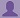SHARE
HELP

To start with, Sr2Jr’s first step is to reduce the expenses related to education. To achieve this goal Sr2Jr organized the textbook’s question and answers. Sr2Jr is community based and need your support to fill the question and answers. The question and answers posted will be available free of cost to all.# Computer Networking : A Top-down Approach

Authors:
James F. Kurose, Keith W. Ross
Exercise:
Problems
Chapter:
Transport Layer
Edition:
6
ISBN:
9780132856201
Question:
46
 Previous Next

###Question

Consider that only a single TCP (Reno) connection uses one 10Mbps link which does not buffer any data. Suppose that this link is the only congested link between the sending and receiving hosts. Assume that the TCP sender has a huge file to send to the receiver, and the receiver’s receive buffer is much larger than the congestion window. We also make the following assumptions: each TCP segment size is 1,500 bytes; the two-way propagation delay of this connection is 150 msec; and this TCP connection is always in congestion avoidance phase, that is, ignore slow start.

a. What is the maximum window size (in segments) that this TCP connection can achieve?

b. What is the average window size (in segments) and average throughput (in bps) of this TCP connection?

c. How long would it take for this TCP connection to reach its maximum window again after recovering from a packet loss?

###Answer

a). The maximum window size:

10^6*0.15/(8*1.5*10^3) = 125. Therefore, the maximum window size is 125 segments.

b). Average window size is  2*W/3 = 84 segments

and average throughput is  84*1500*8/0.15 = 6.72 Mbps

c). It would take 84/2*0.15 = 6.03 sec.

### Discussions

Post the discussion to improve the above solution.